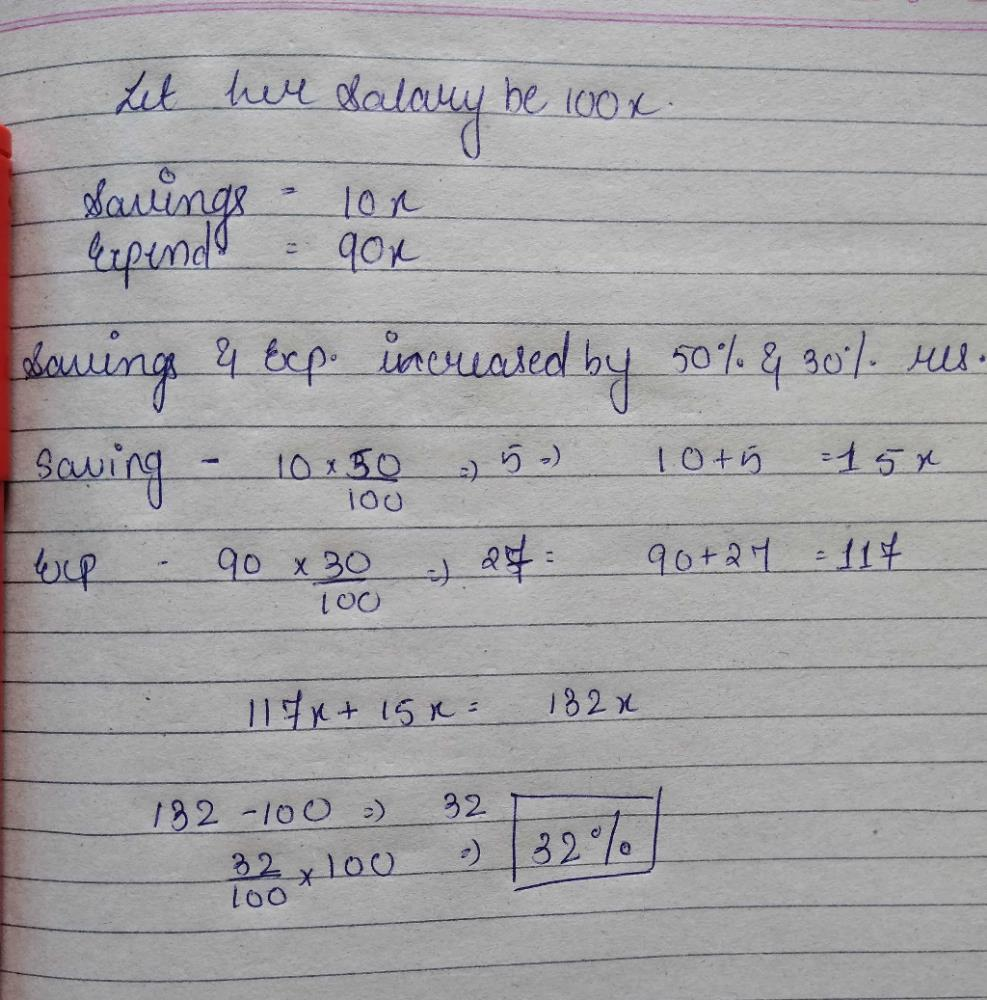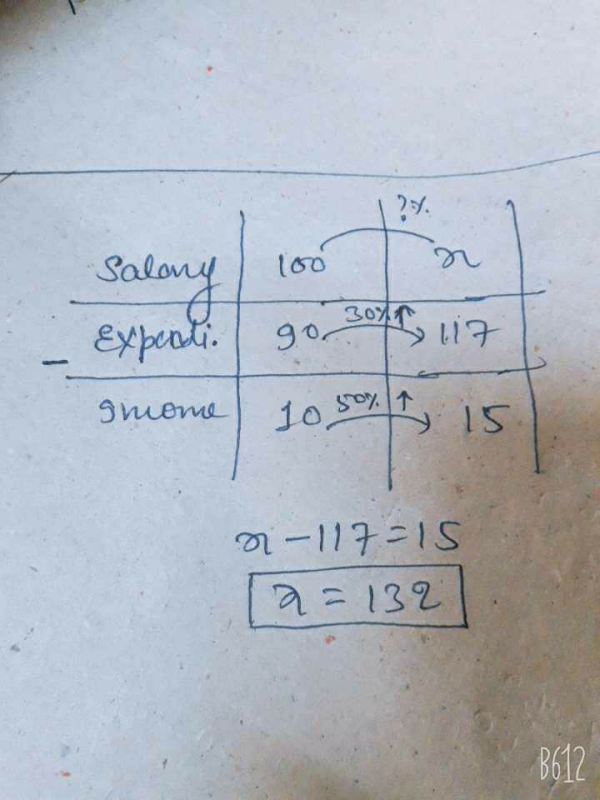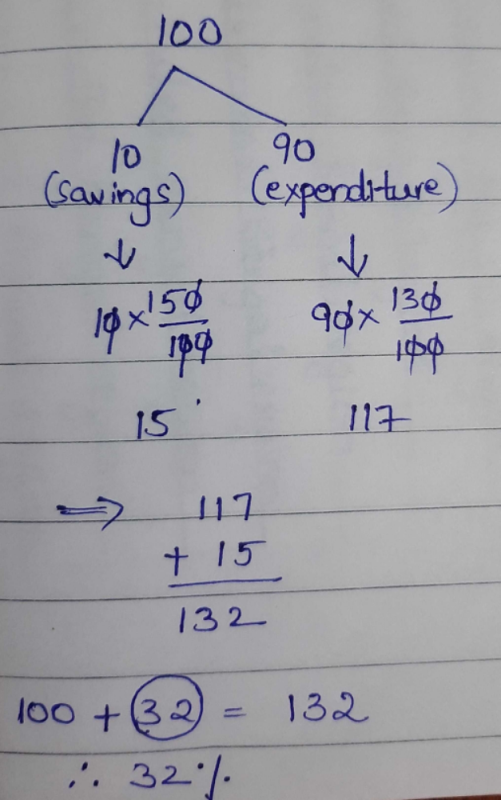Courses

# Suman saves 10% of her monthly salary. Now her expenditure increases by 30% and savings increases by 50%. The present increase in her salary isa)25%b)30%c)32%d)40%Correct answer is option 'C'. Can you explain this answer?

## CLAT Question

By Divya Sharma · Jul 18, 2019 ·CLAT
Aishwarya Rajput answered Feb 08, 2019Let 100Rs=income
then expenditure is 90Rs and saving is 10Rs
now saving is increased by 50%=5Rs
and expenditure by 30%=27Rs
27+5=32.

Prakhya Shrivastava answered Jul 18, 2019
Let's Suman's monthly salary is 100

She saves 10% of her salary
i.e.10% of 100 = 10

Also her expenditure = 100-90 = 90 or 90%

According to the question

When expenditure is increased by 30%
such that, 90+(30% of 90) = 90+[(30/100)×90] = 117

and savings increased by 50%
such that, 10+(50% of 10) = 10+[(50/100)×10) = 15

New salary = expenditure + savings
= 117+15 = 132

Increase in salary = old salary - new salary = 132-100=32

In %age, (32/100)×100 = 32%
Hence option (c) I is correct

Suryakant Verma answered Oct 14, 2019Shanul Haque answered Oct 24, 2019
Let's Suman's monthly salary is 100

She saves 10% of her salary
i.e.10% of 100 = 10

Also her expenditure = 100-90 = 90 or 90%

According to the question

When expenditure is increased by 30%
such that, 90+(30% of 90) = 90+[(30/100)×90] = 117

and savings increased by 50%
such that, 10+(50% of 10) = 10+[(50/100)×10) = 15

New salary = expenditure + savings
= 117+15 = 132

Increase in salary = old salary - new salary = 132-100=32

In %age, (32/100)×100 = 32%
Hence option (c) I is correct

Hema Ambica answered Dec 03, 2019